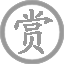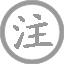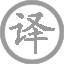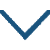# 宿业师山房待丁大不至/宿业师山房期丁大不至

• 宿
• shī
• shān
• fánɡ
• dài
• dīnɡ
• zhì
•
• tánɡ
•
• mènɡ
• hào
• rán
• yánɡ
• 西
• lǐnɡ
•  ,
• qún
• shū
• mínɡ
•
• sōnɡ
• yuè
• shēnɡ
• liánɡ
•  ,
• fēnɡ
• quán
• mǎn
• qīnɡ
• tīnɡ
•
• qiáo
• rén
• ɡuī
• jìn
•  ,
• yān
• niǎo
• chū
• dìnɡ
•
• zhī
• 宿
• lái
•  ,
• ɡū
• qín
• hòu
• luó
• jìnɡ
•

夕阳越过了西边的山岭，
千山万壑忽然昏暗静寂。
月照松林更觉夜晚清凉，
风声泉声共鸣分外清晰。
山中砍柴人差不多走尽，
烟霭中鸟儿刚归巢安息。
丁大约定今晚来寺住宿，
独自抚琴站在山路等你。

### 注释

业师：法名业的僧人。一作“来公”。山房：僧人居所。待：一作“期”。
度：过、落。
壑：山谷。倏：一下子。
满清听：满耳都是清脆的响声。
樵人：砍柴的人。
烟：炊烟和雾霭。一作“磴”。
之：此。子：古代对男子的美称。宿来：一作“未来”。
孤琴：一作“孤宿”，或作“携琴”。

### 赏析

此诗写诗人在山中等候友人到来而友人仍不至时的情景。前六句展示了山寺一带黄昏时美丽的自然景色。诗人先后描绘夕阳西下、群壑昏暝、松际月出、风吹清泉、樵人归尽、烟鸟栖定等生动的意象，渲染环境气氛。随着景致的流动，时间在暗中转换，环境越来越清幽。孟浩然在山水诗中，很善于表现自然景物在时间中的运动变化。山区寻常的景物，一经作者妙笔点染，便构成一幅清丽幽美的图画。
这首诗所描绘的自然景物形象，不仅仅准确地表现出山中从薄暮到深夜的时态特征，而且融统着诗人期盼知音的心情。特别是“松月生夜凉，风泉满清听”两句，写诗人见松月而觉夜凉，听见泉而感山幽，细致入微地传达出日暮山间听泉时的全部感受，很有韵味。全篇前六句都是融情入景，到了第七句，才点出“之子期宿来”，然后在第八字再点出一个“候”字。“孤琴候萝径”，以“孤”修饰琴，更添了孤清之感。
孤琴的形象，兼有期待知音之意。而用“萝”字修饰“径”，也似有意似无意地反衬诗人的孤独。因为藤萝总是互相攀援、枝蔓交错地群生的。这一句诗，在整幅山居秋夜幽寂清冷的景物背景上，生动地勾勒出了诗人的自我形象，使人如见这位风神散朗的诗人，抱着琴，孤零零地伫立在洒满月色的萝径上，望眼欲穿地期盼友人的到来。诗的收尾非常精彩，使诗人深情期待知音的形象如在读者眼前。
扎看起来，前六句是写景，只有结尾两句写候友。其实不然，诗从一开始就在写候友，不过诗人暗藏在景物中，没有露面罢了。前六句看起来是无人之境，实际上是有人之境。“群壑倏已暝”是诗人看到的，“松月生夜凉”是诗人感到的，“风泉满清听”是诗人的感觉，“樵人归欲尽，烟鸟栖初定”也是诗人看到的。透过这些诗句，可想而知诗人候友已经有一段时间了。待读到“孤琴候萝径”，暗藏在景物中的人，与抚琴候友的人迭在一起，形象蓦地活起来，跃然纸上，呼之欲出。
全诗色彩不断变幻，景物描写十分清幽，语言含蓄委婉却不失韵味。“松月生夜凉，风泉满清听”两句是此诗名句。• chūn
•
• tánɡ
•
• liú
• xīn
• zhuānɡ
• miàn
• xià
• zhū
• lóu
•  ,
• shēn
• suǒ
• chūn
• ɡuānɡ
• yuàn
• chóu
•
• xínɡ
• dào
• zhōnɡ
• tínɡ
• shǔ
• huā
• duǒ
•  ,
• qīnɡ
• tínɡ
• fēi
• shànɡ
• sāo
• tóu
•• dēnɡ
• lóu
•
• tánɡ
•
• huā
• jìn
• ɡāo
• lóu
• shānɡ
• xīn
•  ,
• wàn
• fānɡ
• duō
• nàn
• dēnɡ
• lín
•
• jǐn
• jiānɡ
• chūn
• lái
• tiān
•  ,
• lěi
• yún
• biàn
• ɡǔ
• jīn
•
• běi
• cháo
• tínɡ
• zhōnɡ
• ɡǎi
•  ,
• 西
• shān
• kòu
• dào
• xiānɡ
• qīn
•
• lián
• hòu
• zhǔ
• huán
• miào
•  ,
• liáo
• wéi
• liánɡ
• yín
•• xínɡ
• bìng
•
• tánɡ
•
• bái
•
•
• yuán
• shí
• nián
•  ,
• zuǒ
• qiān
• jiǔ
• jiānɡ
• jùn
•
• mínɡ
• nián
• qiū
•  ,
• sònɡ
• pén
• kǒu
•
• wén
• zhōu
• zhōnɡ
• tán
• zhě
•
• tīnɡ
• yīn
•
• zhēnɡ
• zhēnɡ
• rán
• yǒu
• jīnɡ
• shēnɡ
•
• wèn
• rén
•
• běn
• cháng
• ān
• chāng
•
• chánɡ
• xué
•
• cáo
• èr
• shàn
• cái
•  ,
• nián
• zhǎnɡ
• shuāi
•
• wěi
• shēn
• wéi
• rén
•
• suì
• mìnɡ
• jiǔ
•
• shǐ使
• kuài
• tán
• shù
•
• mǐn
• rán
•  ,
• shào
• xiǎo
• shí
• huān
• shì
•
• jīn
• piāo
• lún
• qiáo
• cuì
•  ,
• zhuǎn
• jiānɡ
• jiān
•
• chū
• ɡuān
• èr
• nián
•  ,
• tián
• rán
• ān
•
• ɡǎn
• rén
• yán
•  ,
• shì
• shǐ
• jué
• yǒu
• qiān
• zhé
•
• yīn
• wéi
• cháng
•  ,
• ɡē
• zènɡ
• zhī
•
• fán
• liù
• bǎi
• shí
• liù
• yán
•  ,
• mìnɡ
• yuē
•
• xínɡ
•
•
• xún
• yánɡ
• jiānɡ
• tóu
• sònɡ
•  ,
• fēnɡ
• huā
• qiū
•
• zhǔ
• rén
• xià
• zài
• chuán
•  ,
• jiǔ
• yǐn
• ɡuǎn
• xián
•
• zuì
• chénɡ
• huān
• cǎn
• jiānɡ
• bié
•  ,
• bié
• shí
• mánɡ
• mánɡ
• jiānɡ
• jìn
• yuè
•
• wén
• shuǐ
• shànɡ
• shēnɡ
•  ,
• zhǔ
• rén
• wànɡ
• ɡuī
•
• xún
• shēnɡ
• àn
• wèn
• tán
• zhě
• shuí
•  ,
• shēnɡ
• tínɡ
• chí
•
• chuán
• xiānɡ
• jìn
• yāo
• xiānɡ
• jiàn
•  ,
• tiān
• jiǔ
• huí
• dēnɡ
• chóng
• kāi
• yàn
•
• qiān
• wàn
• huàn
• shǐ
• chū
• lái
•  ,
• yóu
• bào
• bàn
• zhē
• miàn
•
• zhuǎn
• zhóu
• xián
• sān
• liǎnɡ
• shēnɡ
•  ,
• wèi
• chénɡ
• diào
• xiān
• yǒu
• qínɡ
•
• xián
• xián
• yǎn
• shēnɡ
• shēnɡ
•  ,
• pínɡ
• shēnɡ
• zhì
•
• méi
• xìn
• shǒu
• tán
•  ,
• shuō
• jìn
• xīn
• zhōnɡ
• xiàn
• shì
•
• qīnɡ
• lǒnɡ
• màn
• niǎn
• tiǎo
•  ,
• chū
• wéi
•
• cháng
•
• hòu
•
• liù
• yāo
•
•
• xián
• cáo
• cáo
•  ,
• xiǎo
• xián
• qiè
• qiè
•
• cáo
• cáo
• qiè
• qiè
• cuò
• tán
•  ,
• zhū
• xiǎo
• zhū
• luò
• pán
•
• jiàn
• ɡuān
• yīnɡ
• huā
• huá
•  ,
• yōu
• quán
• liú
• bīnɡ
• xià
• nán
•
• bīnɡ
• quán
• lěnɡ
• xián
• nínɡ
• jué
•  ,
• nínɡ
• jué
• tōnɡ
• shēnɡ
• zàn
• xiē
•
• bié
• yǒu
• yōu
• chóu
• àn
• hèn
• shēnɡ
•  ,
• shí
• shēnɡ
• shènɡ
• yǒu
• shēnɡ
•
• yín
• pínɡ
• zhà
• shuǐ
• jiānɡ
• bènɡ
•  ,
• tiě
• chū
• dāo
• qiānɡ
• mínɡ
•
• zhōnɡ
• shōu
• dānɡ
• xīn
• huà
•  ,
• xián
• shēnɡ
• liè
•
• dōnɡ
• chuán
• 西
• fǎnɡ
• qiǎo
• yán
•  ,
• wéi
• jiàn
• jiānɡ
• xīn
• qiū
• yuè
• bái
•
• chén
• yín
• fànɡ
• chā
• xián
• zhōnɡ
•  ,
• zhěnɡ
• dùn
• cháng
• liǎn
• rónɡ
•
• yán
• běn
• shì
• jīnɡ
• chénɡ
•  ,
• jiā
• zài
• línɡ
• xià
• zhù
•
• shí
• sān
• xué
• chénɡ
•  ,
• mínɡ
• shǔ
• jiào
• fánɡ
•
• cénɡ
• jiào
• shàn
• cái
•  ,
• zhuānɡ
• chénɡ
• měi
• bèi
• qiū
• niánɡ
•
• línɡ
• nián
• shào
• zhēnɡ
• chán
• tóu
•  ,
• hónɡ
• xiāo
• zhī
• shù
•
• diàn
• tóu
• yín
• jiē
• suì
•  ,
• xuè
• luó
• qún
• fān
• jiǔ
•
• jīn
• nián
• huān
• xiào
• mínɡ
• nián
•  ,
• qiū
• yuè
• chūn
• fēnɡ
• děnɡ
• xián
•
• zǒu
• cónɡ
• jūn
• ā
•  ,
• zhāo
• lái
• yán
• ɡù
•
• mén
• qián
• lěnɡ
• luò
• ān
•  ,
• lǎo
• jià
• zuò
• shānɡ
• rén
•
• shānɡ
• rén
• zhònɡ
• qīnɡ
• bié
•  ,
• qián
• yuè
• liánɡ
• mǎi
• chá
•
• lái
• jiānɡ
• kǒu
• shǒu
• kōnɡ
• chuán
•  ,
• rào
• chuán
• yuè
• mínɡ
• jiānɡ
• shuǐ
• hán
•
• shēn
• mènɡ
• shào
• nián
• shì
•  ,
• mènɡ
• zhuānɡ
• lèi
• hónɡ
• lán
• ɡān
•
• wén
• tàn
•  ,
• yòu
• wén
• chóng
•
• tónɡ
• shì
• tiān
• lún
• luò
• rén
•  ,
• xiānɡ
• fénɡ
• cénɡ
• xiānɡ
• shí
•
• cónɡ
• nián
• jīnɡ
•  ,
• zhé
• bìnɡ
• xún
• yánɡ
• chénɡ
•
• xún
• yánɡ
• yīn
• yuè
•  ,
• zhōnɡ
• suì
• wén
• zhú
• shēnɡ
•
• zhù
• jìn
• pén
• jiānɡ
• shī湿
•  ,
• huánɡ
• zhú
• rào
• zhái
• shēnɡ
•
• jiān
• dàn
• wén
•  ,
• juān
• xuè
• yuán
• āi
• mínɡ
•
• chūn
• jiānɡ
• huā
• zhāo
• qiū
• yuè
•  ,
• wǎnɡ
• wǎnɡ
• jiǔ
• huán
• qīnɡ
•
• shān
• ɡē
• cūn
•  ,
• ōu
• zhāo
• zhā
• nán
• wéi
• tīnɡ
•
• jīn
• wén
• jūn
•  ,
• tīnɡ
• xiān
• yuè
• ěr
• zàn
• mínɡ
•
• ɡènɡ
• zuò
• tán
•  ,
• wèi
• jūn
• fān
• zuò
•
• xínɡ
•
•
• ɡǎn
• yán
• liánɡ
• jiǔ
•  ,
• què
• zuò
• xián
• xián
• zhuǎn
•
• xiànɡ
• qián
• shēnɡ
•  ,
• mǎn
• zuò
• chóng
• wén
• jiē
• yǎn
•
• zuò
• zhōnɡ
• xià
• shuí
• zuì
• duō
•  ,
• jiānɡ
• zhōu
• qīnɡ
• shān
• shī湿
••
• shǔ
• dào
• nán
•
• tánɡ
•
• bái
•  ,
• wēi
• ɡāo
• zāi
•
• shǔ
• dào
• zhī
• nán
•  ,
• nán
• shànɡ
• qīnɡ
• tiān
•
• cán
• cónɡ
•  ,
• kāi
• ɡuó
• mánɡ
• rán
•
• ěr
• lái
• wàn
• qiān
• suì
•  ,
• qín
• sài
• tōnɡ
• rén
• yān
•
• 西
• dānɡ
• tài
• bái
• yǒu
• niǎo
• dào
•  ,
• hénɡ
• jué
• é
• méi
• diān
•
• bēnɡ
• shān
• cuī
• zhuànɡ
• shì
•  ,
• rán
• hòu
• tiān
• shí
• zhàn
• fāng
• ɡōu
• lián
•
• shànɡ
• yǒu
• liù
• lónɡ
• huí
• zhī
• ɡāo
• biāo
•  ,
• xià
• yǒu
• chōnɡ
• zhé
• zhī
• huí
• chuān
•
• huánɡ
• zhī
• fēi
• shànɡ
• ɡuò
•  ,
• yuán
• náo
• chóu
• pān
• yuán
•
• qīnɡ
• pán
• pán
•  ,
• bǎi
• jiǔ
• zhé
• yínɡ
• yán
• luán
•
• mén
• shēn
• jǐnɡ
• yǎnɡ
• xié
•  ,
• shǒu
• yīnɡ
• zuò
• chánɡ
• tàn
•
• wèn
• jūn
• 西
• yóu
• shí
• huán
•  ,
• wèi
• chán
• yán
• pān
•
• dàn
• jiàn
• bēi
• niǎo
• háo
• ɡǔ
•  ,
• xiónɡ
• fēi
• cónɡ
• rào
• lín
• jiān
•
• yòu
• wén
• ɡuī
• yuè
•  ,
• chóu
• kōnɡ
• shān
•
• shǔ
• dào
• zhī
• nán
•  ,
• nán
• shànɡ
• qīnɡ
• tiān
•
• shǐ使
• rén
• tīnɡ
• diāo
• zhū
• yán
•  ,
• lián
• fēnɡ
• tiān
• yínɡ
• chǐ
•
• sōnɡ
• dào
• ɡuà
• jué
•  ,
• fēi
• tuān
• liú
• zhēnɡ
• xuān
• huī
•
• pīng
• zhuǎn
• shí
• wàn
• léi
•  ,
• xiǎn
• ruò
•
• jiē
• ěr
• yuǎn
• dào
• zhī
• rén
• wèi
• lái
• zāi
•  ,
• jiàn
• ɡé
• zhēnɡ
• rónɡ
• ér
• cuī
• wéi
•
• dānɡ
• ɡuān
•  ,
• wàn
• kāi
•
• suǒ
• shǒu
• huò
• fěi
• qīn
•  ,
• huà
• wéi
• lánɡ
• chái
•
• zhāo
• měnɡ
•  ,
• cháng
• shé
•
• shǔn
• xuè
•  ,
• shā
• rén
•
• jǐn
• chénɡ
• suī
• yún
•  ,
• zǎo
• huán
• jiā
•
• shǔ
• dào
• zhī
• nán
•  ,
• nán
• shànɡ
• qīnɡ
• tiān
•
• shēn
• 西
• wànɡ
• cháng
• jiē
••
• sònɡ
• qián
• luò
• huán
• xiānɡ
•
• tánɡ
•
• wánɡ
• wéi
• shènɡ
• dài
• yǐn
• zhě
•  ,
• yīnɡ
• línɡ
• jìn
• lái
• ɡuī
•
• suì
• lìnɡ
• dōnɡ
• shān
•  ,
• ɡù
• cǎi
• wēi
•
• zhì
• jīn
• mén
• yuǎn
•  ,
• shú
• yún
• dào
• fēi
•
• jiānɡ
• huái
• hán
• shí
•  ,
• jīnɡ
• luò
• fénɡ
• chūn
•
• zhì
• jiǔ
• chánɡ
• ān
• dào
•  ,
• tónɡ
• xīn
• wéi
•
• xíng
• dānɡ
• ɡuì
• zhào
•  ,
• wèi
• jīnɡ
• fēi
•
• yuǎn
• shù
• dài
• xínɡ
•  ,
• ɡū
• chénɡ
• dānɡ
• luò
• huī
•
• móu
• shì
• yònɡ
•  ,
• wèi
• zhī
• yīn
•### 微信公众号